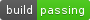2016/01/11 08:28

# Numexpr: Fast numerical expression evaluator for NumPy

Author: David M. Cooke, Francesc Alted and others faltet@gmail.com https://github.com/pydata/numexpr|version|

## What it is Numexpr?

Numexpr is a fast numerical expression evaluator for NumPy.  With it, expressions that operate on arrays (like "3*a+4*b") are accelerated and use less memory than doing the same calculation in Python.

In addition, its multi-threaded capabilities can make use of all your cores, which may accelerate computations, most specially if they are not memory-bounded (e.g. those using transcendental functions).

Last but not least, numexpr can make use of Intel's VML (Vector Math Library, normally integrated in its Math Kernel Library, or MKL). This allows further acceleration of transcendent expressions.

## Examples of use

>>> import numpy as np
>>> import numexpr as ne

>>> a = np.arange(1e6)   # Choose large arrays for better speedups
>>> b = np.arange(1e6)

>>> ne.evaluate("a + 1")   # a simple expression
array([  1.00000000e+00,   2.00000000e+00,   3.00000000e+00, ...,
9.99998000e+05,   9.99999000e+05,   1.00000000e+06])

>>> ne.evaluate('a*b-4.1*a > 2.5*b')   # a more complex one
array([False, False, False, ...,  True,  True,  True], dtype=bool)

>>> ne.evaluate("sin(a) + arcsinh(a/b)")   # you can also use functions
array([        NaN,  1.72284457,  1.79067101, ...,  1.09567006,
0.17523598, -0.09597844])

>>> s = np.array(['abba', 'abbb', 'abbcdef'])
>>> ne.evaluate("'abba' == s")   # string arrays are supported too
array([ True, False, False], dtype=bool)

## Datatypes supported internally

Numexpr operates internally only with the following types:

* 8-bit boolean (bool)
* 32-bit signed integer (int or int32)
* 64-bit signed integer (long or int64)
* 32-bit single-precision floating point number (float or float32)
* 64-bit, double-precision floating point number (double or float64)
* 2x64-bit, double-precision complex number (complex or complex128)
* Raw string of bytes (str)

If the arrays in the expression does not match any of these types, they will be upcasted to one of the above types (following the usual type inference rules, see below).  Have this in mind when doing estimations about the memory consumption during the computation of your expressions.

Also, the types in Numexpr conditions are somewhat stricter than those of Python.  For instance, the only valid constants for booleans are True and False, and they are never automatically cast to integers.

## Casting rules

Casting rules in Numexpr follow closely those of NumPy.  However, for implementation reasons, there are some known exceptions to this rule, namely:

* When an array with type int8, uint8, int16 or uint16 is
used inside Numexpr, it is internally upcasted to an int (or
int32 in NumPy notation).

* When an array with type uint32 is used inside Numexpr, it is
internally upcasted to a long (or int64 in NumPy notation).

* A floating point function (e.g. sin) acting on int8 or
int16 types returns a float64 type, instead of the float32
that is returned by NumPy functions.  This is mainly due to the
absence of native int8 or int16 types in Numexpr.

* In operations implying a scalar and an array, the normal rules
of casting are used in Numexpr, in contrast with NumPy, where
array types takes priority.  For example, if 'a' is an array of
type float32 and 'b' is an scalar of type float64 (or Python
float type, which is equivalent), then 'a*b' returns a
float64 in Numexpr, but a float32 in NumPy (i.e. array
operands take priority in determining the result type).  If you
need to keep the result a float32, be sure you use a float32
scalar too.

## Supported operators

Numexpr supports the set of operators listed below:

* Logical operators: &, |, ~
* Comparison operators: <, <=, ==, !=, >=, >
* Unary arithmetic operators: -
* Binary arithmetic operators: +, -, *, /, **, %, <<, >>

## Supported functions

The next are the current supported set:

* where(bool, number1, number2): number
Number1 if the bool condition is true, number2 otherwise.
* {sin,cos,tan}(float|complex): float|complex
Trigonometric sine, cosine or tangent.
* {arcsin,arccos,arctan}(float|complex): float|complex
Trigonometric inverse sine, cosine or tangent.
* arctan2(float1, float2): float
Trigonometric inverse tangent of float1/float2.
* {sinh,cosh,tanh}(float|complex): float|complex
Hyperbolic sine, cosine or tangent.
* {arcsinh,arccosh,arctanh}(float|complex): float|complex
Hyperbolic inverse sine, cosine or tangent.
* {log,log10,log1p}(float|complex): float|complex
Natural, base-10 and log(1+x) logarithms.
* {exp,expm1}(float|complex): float|complex
Exponential and exponential minus one.
* sqrt(float|complex): float|complex
Square root.
* abs(float|complex): float|complex
Absolute value.
* conj(complex): complex
Conjugate value.
* {real,imag}(complex): float
Real or imaginary part of complex.
* complex(float, float): complex
Complex from real and imaginary parts.
* contains(str, str): bool
Returns True for every string in op1 that contains op2.

More functions can be added if you need them.

## Supported reduction operations

The next are the current supported set:

• sum(number, axis=None): Sum of array elements over a given axis. Negative axis are not supported.

• prod(number, axis=None): Product of array elements over a given axis.  Negative axis are not supported.

## General routines

* evaluate(expression, local_dict=None, global_dict=None,
out=None, order='K', casting='safe', **kwargs):
Evaluate a simple array expression element-wise.  See docstrings
for more info on parameters.  Also, see examples above.

* test():  Run all the tests in the test suite.

* print_versions():  Print the versions of software that numexpr
relies on.

operations.  Returns the previous setting for the number of
threads.  During initialization time Numexpr sets this number to
the number of detected cores in the system (see
detect_number_of_cores()).

If you are using Intel's VML, you may want to use
set_vml_num_threads(nthreads) to perform the parallel job with
VML instead.  However, you should get very similar performance
with VML-optimized functions, and VML's parallelizer cannot deal
with common expresions like (x+1)*(x-2), while Numexpr's one
can.

* detect_number_of_cores(): Detects the number of cores in the
system.

## Intel's VML specific support routines

When compiled with Intel's VML (Vector Math Library), you will be able to use some additional functions for controlling its use. These are:

• set_vml_accuracy_mode(mode):  Set the accuracy for VML operations.

• The mode parameter can take the values:

• 'low': Equivalent to VML_LA - low accuracy VML functions are called

• 'high': Equivalent to VML_HA - high accuracy VML functions are called

• 'fast': Equivalent to VML_EP - enhanced performance VML functions are called

It returns the previous mode.

This call is equivalent to the vmlSetMode() in the VML library. See:

http://www.intel.com/software/products/mkl/docs/webhelp/vml/vml_DataTypesAccuracyModes.html

This function is equivalent to the call mkl_domain_set_num_threads(nthreads, MKL_DOMAIN_VML) in the MKL library. See:

• get_vml_version():  Get the VML/MKL library version.

## How Numexpr can achieve such a high performance?

The main reason why Numexpr achieves better performance than NumPy (or even than plain C code) is that it avoids the creation of whole temporaries for keeping intermediate results, so saving memory bandwidth (the main bottleneck in many computations in nowadays computers). Due to this, it works best with arrays that are large enough (typically larger than processor caches).

Briefly, it works as follows. Numexpr parses the expression into its own op-codes, that will be used by the integrated computing virtual machine. Then, the array operands are split in small chunks (that easily fit in the cache of the CPU) and passed to the virtual machine. Then, the computational phase starts, and the virtual machine applies the op-code operations for each chunk, saving the outcome in the resulting array. It is worth noting that all the temporaries and constants in the expression are kept in the same small chunk sizes than the operand ones, avoiding additional memory (and most specially, memory bandwidth) waste.

The result is that Numexpr can get the most of your machine computing capabilities for array-wise computations.  Just to give you an idea of its performance, common speed-ups with regard to NumPy are usually between 0.95x (for very simple expressions, like ’a + 1’) and 4x (for relatively complex ones, like 'a*b-4.1*a > 2.5*b'), although much higher speed-ups can be achieved (up to 15x can be seen in not too esoteric expressions) because this depends on the kind of the operations and how many operands participates in the expression.  Of course, Numexpr will perform better (in comparison with NumPy) with larger matrices, i.e. typically those that does not fit in the cache of your CPU.  In order to get a better idea on the different speed-ups that can be achieved for your own platform, you may want to run the benchmarks in the directory bench/.

https://github.com/pydata/numexpr/wiki

## Authors

See AUTHORS.txt### 作者的其它热门文章

1
0 收藏

0 评论
0 收藏
1